# Precalculus : Divide Polynomials by Binomials Using Synthetic Division

## Example Questions

### Example Question #1 : Divide Polynomials By Binomials Using Synthetic Division

Divide the polynomial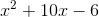by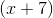.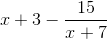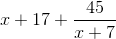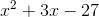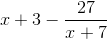Explanation:

Our first step is to list the coefficients of the polynomials in descending order and carry down the first coefficient.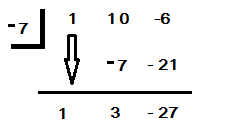We multiply what's below the line byand place the product on top of the line. We find the sum of this number with the next coefficient and place the sum below the line. We keep repeating these steps until we've reached the last coefficients.

To write the answer, we use the numbers below the line as our new coefficients. The last number is our remainder.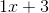with remainder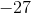This can be rewritten asKeep in mind: the highest degree of our new polynomial will always be one less than the degree of the original polynomial.

### Example Question #2 : Divide Polynomials By Binomials Using Synthetic Division

Divide the polynomial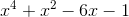by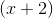.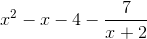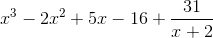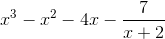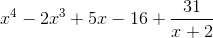Explanation:

Our first step is to list the coefficients of the polynomials in descending order and carry down the first coefficient.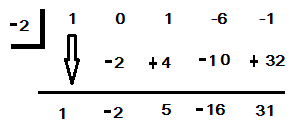We multiply what's below the line byand place the product on top of the line. We find the sum of this number with the next coefficient and place the sum below the line. We keep repeating these steps until we've reached the last coefficients.

To write the answer, we use the numbers below the line as our new coefficients. The last number is our remainder.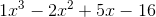with remainderThis can be rewritten as:Keep in mind: the highest degree of our new polynomial will always be one less than the degree of the original polynomial.

### Example Question #3 : Divide Polynomials By Binomials Using Synthetic Division

Divide the polynomial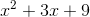by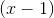.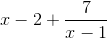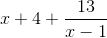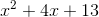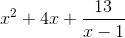Explanation:

Our first step is to list the coefficients of the polynomials in descending order and carry down the first coefficient.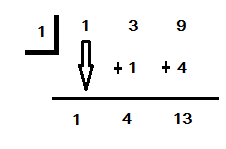We mulitply what's below the line by 1 and place the product on top of the line. We find the sum of this number with the next coefficient and place the sum below the line. We keep repeating these steps until we've reached the last coefficients.

To write the answer, we use the numbers below the line as our new coefficients. The last number is our remainder.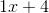with remainderKeep in mind: the highest degree of our new polynomial will always be one less than the degree of the original polynomial.

### Example Question #4 : Divide Polynomials By Binomials Using Synthetic Division

Divide the polynomial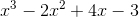by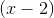.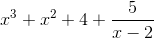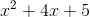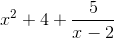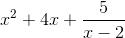Explanation:

Our first step is to list the coefficiens of the polynomials in descending order and carry down the first coefficient.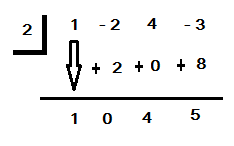We multiply what's below the line byand place the product on top of the line. We find the sum of this number with the next coefficient and place the sum below the line. We keep repeating these steps until we've reached the last coefficient.

To write the answer, we use the numbers below the line as our new coefficients. The last number is our remainder.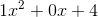with reminderThis can be rewritten as:### Example Question #5 : Divide Polynomials By Binomials Using Synthetic Division

Divide the polynomial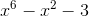by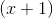.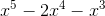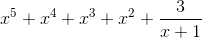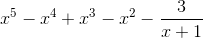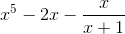Explanation:

Our first step is to list the coefficients of the polynomials in descending order and carry down the first coefficient.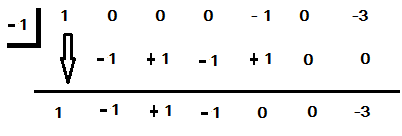Remember to place awhen there isn't a coefficient given.

We multiply what's below the line byand place the product on top of the line. We find the sum of this number with the next coefficient and place the sum below the line. We keep repeating these steps until we've reached the last coefficients.

To write the answer, we use the numbers below the line as our new coefficients. The last number is our remainder.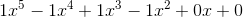with remainderThis can be rewritten as:Keep in mind: the highest degree of our new polynomial will always be one less than the degree of the original polynomial.

### Example Question #6 : Divide Polynomials By Binomials Using Synthetic Division

Use synthetic division to divide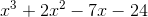by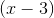.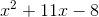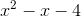Remainder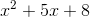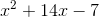Remainder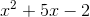Remainder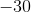Explanation:

To divide synthetically, we begin by drawing a box. On the inside separated by spaces, we write the coefficients of the terms of our polynomial being divided. On the outside, we write the root that would satisfy our binomial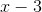, namely.  Leaving a space for another row of numbers, we then draw a line below our row of coefficients.

We then begin dividing by simply carrying our first coefficient (1) down below the line.

We then multiply this 1 by our divisor (3) and write the resulting product (3) below our next coefficient.

We then add the two numbers in that column and write the sum (5) below the line.

We then simply continue the process by multiplying this 5 by our divisor 3 and writing that product in the next column, adding it to the next coefficient, and continuing until we finish the columns.

We then need to translate our bottom row of numbers into the coefficients of our new quotient. Since the first column originally corresponded to our cubic term, it will now correspond to the quadratic term meaning that our 1 can be translated as.  Similarly, our second column transitions from quadratic to linear, making our 5 become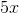.  Finally, our third column becomes the constant term, meaning 8 simply remains the constant 8.  Finally, our former constant column becomes the column for our remainder.  However, since we have a 0, we have no remainder and can disregard it.

Putting all of this together gives us a final answer of### Example Question #7 : Divide Polynomials By Binomials Using Synthetic Division

Divide using synthetic division: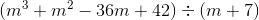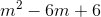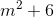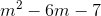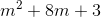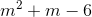Explanation:

First, set up the synthetic division problem by lining up the coefficients. There are a couple of different strategies - for this one, we will put a -7 in the top corner and add the columns.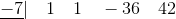_________________________

The first step is to bring down the first 1. Then multiply what is below the line by the -7 in the box, write it below the next coefficient, and then add the columns: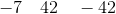_________________________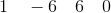We can interpret this answer as meaning### All Precalculus Resources